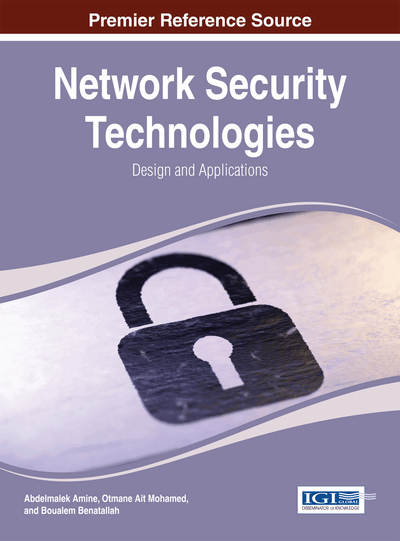# Formal Reliability Analysis of Engineering Systems

DOI: 10.4018/978-1-4666-4789-3.ch013
Available
\$37.50
No Current Special Offers

## Abstract

Reliability analysis of engineering systems has traditionally been done using computationally expensive computer simulations that cannot attain 100% accuracy due to their inherent limitations. The authors conduct a formal reliability analysis using higher-order-logic theorem proving, which is known to be sound, accurate, and exhaustive. For this purpose, they present the higher-order-logic formalization of independent multiple continuous random variables, their verified probabilistic properties, and generalized relations for commonly encountered reliability structures in engineering systems. To illustrate the usefulness of the approach, the authors present the formal reliability analysis of a single stage transmission of an automobile.
Chapter Preview
Top

## 1. Introduction

The reliability of an engineering system is very important as an unreliable system usually translates to loss of both money and time and a considerable amount of inconvenience. Such reliability analysis is conducted using probabilistic techniques while considering the individual reliabilities of sub-components of the given engineering systems. This analysis usually involves building a model of the given engineering system using random variables and various continuous physical parameters. Computer simulations have traditionally been used for the reliability analysis of engineering systems. Computer simulations are automatic and thus user friendly and can be used to analyze analytically complex systems including the ones that cannot be modeled in a closed mathematical form. However, they cannot guarantee 100% accurate results, because 1) infinite precision real numbers, corresponding to the physical parameters of the system, cannot be precisely modelled in computer memory, 2) due to the enormous size of the present-age engineering systems, e.g., a modern power plant is composed of over a million components, exhaustive testing of all possible input scenarios is not possible due to limited computational resources, and 3) random variables are usually approximated using pseudo random number generators that are not truly random. The accuracy of reliability analysis of engineering systems has become a dire need these days due to their extensive usage in safety-critical applications where an incorrect reliability estimate may lead to disastrous situations including the loss of innocent lives.

Formal methods based techniques such as probabilistic model checking (Baier, Haverkort, Hermanns, & Katoen, 2003) and probabilistic theorem proving (Hasan, 2008) can alleviate some of the inaccuracy limitations of computer simulations. Model checking based techniques can handle finite sized systems or finite models of infinite systems. Some basic probabilistic and statistical reliability properties of an engineering system can be verified using this technique. However, such results cannot be considered truly formal as the decision procedures used in this process depend on numerical computations.

Theorem proving based techniques, on the other hand, do not suffer from these limitations, however, they lack the foundational formalization required for the reliability analysis of engineering systems. This includes formal reasoning support for multiple independent continuous random variables and relations that describe the reliability of engineering systems in terms of their sub-components.

This paper is targeted towards developing these foundations to facilitate formal reliability analysis using theorem proving. The proposed reliability analysis framework is shown in Figure 1. The reliability modeling and analysis process begins with the construction of a formal model of the system and its environment. The functional and reliability requirements of the system are then formally stated. The proposed reliability analysis framework then facilitates verification, computation, reasoning, and documentation of the reliability proofs in the sound environment of the HOL theorem prover. Finally, the formal functional and reliability analysis results are unformalized and interpreted and stated in an appropriate language in the problem domain.

The two main contributions of this paper are that: 1) it presents the formalization of multiple independent continuous random variables, and the verification of the standard cumulative distribution function properties of multiple continuous random variables in the sound core of the HOL theorem prover; 2) it presents the formalization of various commonly used reliability structures such as series, parallel, series-parallel and parallel-series in higher-order logic. These contributions play a vital role in conducting formal reliability analysis as the multiple continuous random variables can be used to model the randomness associated with each sub component of an engineering system while the reliability structure related formalization can be utilized to construct formal models of the given system using its sub-components as modules and reasoning about its associated properties.

## Complete Chapter List

Search this Book:
Reset